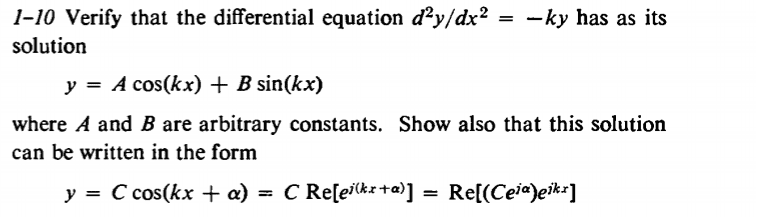# Differential Equation, Rewriting solution

## Homework Statement(also express C and alpha as functions of A and B)

I need help with the second part (rewriting the solution).

## Homework Equations

e = cos(θ) + jsin(θ)

## The Attempt at a Solution

Unfortunately, I can't think of how to even begin solving. I have the notion that I have to combine the two terms into one, however the A and B constants prevent me from doing so. Can someone point me in the right direction?

Thanks

#### Attachments

Chestermiller
Mentor
Have you been able to do the first part?

Have you been able to do the first part?

Yes, I have already done the first part. I did do it backwards, however, because I do not know how to solve differential equations (second derivative ---> function) yet.

Chestermiller
Mentor
Yes, I have already done the first part. I did do it backwards, however, because I do not know how to solve differential equations (second derivative ---> function) yet.
Then by the same backwards approach, do you know the trigonometric identity for the cosine of the sum of two angles?

•ecoo
Then by the same backwards approach, do you know the trigonometric identity for the cosine of the sum of two angles?

Thanks for the help